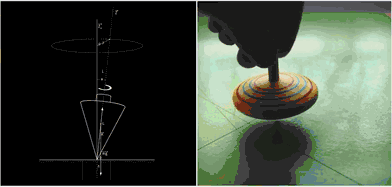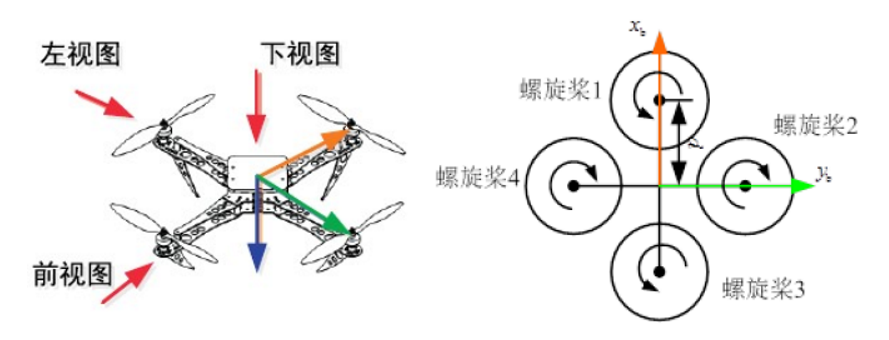# [飞控] 建模进阶 (一)-陀螺力矩$$\boldsymbol { M } _ { g y r o } = \boldsymbol { J_{RP} \Omega } \times \boldsymbol { \omega }$$

$$\Omega$$

$$\omega$$

（我们可以认为转轴的角速度等于机体角速度）

$$J_{RP} \Omega_k \boldsymbol{b_3} \times \boldsymbol{\omega}$$

$$\boldsymbol{b_3} \times \boldsymbol{ \omega } = - \boldsymbol{ \omega } \times \boldsymbol{b_3}$$

$$\boldsymbol { M } _ { g y r o } = \boldsymbol { J_{RP} \omega } \times \boldsymbol { \Omega } = -J_{RP} (\boldsymbol{ \omega } \times \boldsymbol {b_3} ) \Omega_{k}$$

$$\left[ \begin{array} { c } { \omega _ { x } } \\ { \omega _ { y } } \\ { \omega _ { z } } \end{array} \right] \iff \left[ \begin{array} { c c c } { 0 } & { - w_z } & { w_y } \\ { w_z } & { 0 } & { - w_x } \\ { - w_y } & { w_x } & { 0 } \end{array} \right] = \omega ^ \lor$$

\begin{aligned}\boldsymbol{ \omega } \times \boldsymbol{b_3} & = \boldsymbol { \omega ^ \lor } \boldsymbol{b_3} \\ &= \left[ \begin{array} { c c c } { 0 } & { - w_z } & { w_y } \\ { w_z } & { 0 } & { - w_x } \\ { - w_y } & { w_x } & { 0 } \end{array} \right] \left[ \begin{array} { c } { 0 } \\ { 0 } \\ { 1 } \end{array} \right] \\ &= \left[ \begin{array} { c } { w_y } \\ { -w_x } \\ { 0 } \end{array} \right] \\ \end{aligned}

$$\boldsymbol { M } _ { g y r o } = - J_{RP} \Omega_{k}\left[ \begin{array} { c } { w_y } \\ { -w_x } \\ { 0 } \end{array} \right]$$$$\Omega_{k}=-\Omega_{1}\\$$

$$\left\{ \begin{array} { l } { \tau _ { x } ^ { \prime } = J _ { RP } \omega _ { y } \left( \Omega _ { 1 } - \Omega _ { 2 } + \Omega _ { 3 } - \Omega _ { 4 } \right) } \\ { \tau _ { y } ^ { \prime } = J _ { RP } \omega _ { x } \left( -\Omega _ { 1 } + \Omega _ { 2 } - \Omega _ { 3 } + \Omega _ { 4 } \right) } \\ {\tau _ { z } = 0} \end{array} \right.$$